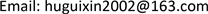﻿ 一阶常微分方程通解中任意常数的分析及教学 The Analysis and Teaching of the Arbitrary Constant in the Usual Solution of First Order Ordinary Differential Equation

Vol.06 No.07(2017), Article ID:22541,4 pages
10.12677/AAM.2017.67103

The Analysis and Teaching of the Arbitrary Constant in the Usual Solution of First Order Ordinary Differential Equation

Guixin Hu

School of Mathematics and Information Science, Henan Polytechnic University (HPU), Jiaozuo HenanReceived: Oct. 8th, 2017; accepted: Oct. 24th, 2017; published: Oct. 31st, 2017ABSTRACT

The arbitrary constant of the usual solution to first order ordinary differential equation is discussed in this paper. Firstly, the arbitrary constant in the usual solution does not necessarily take over all of the real numbers. Secondly, the arbitrary constant and special solution is not necessarily a one-to-one correspondence, that is, a constant does not determine a unique special solution, also is not that each special solution can be determined by the arbitrary constant in usual solution. In order to use the method of variation of constant to solve the first order linear differential equation, the canonical form of the usual solution to the homogeneous equation of first order linear differential equation is provided.

Keywords:Arbitrary Constant, Usual Solution, Ordinary Differential Equation1. 引言

2. 主要结果

2.1. 任意常数C不一定取遍所有的实数

$\left({\text{e}}^{x}+C\right){\text{e}}^{y}+1=0$ (1)

2.2. 任意常数和方程的特解不一定是一对一的

2.2.1. 一个固定的C不一定仅确定一个特解

$\mathrm{arcsin}y=\mathrm{arcsin}x+C,\text{\hspace{0.17em}}\text{\hspace{0.17em}}-\text{π} (2)

${y}^{2}+{x}^{2}-2xy\mathrm{cos}C={\mathrm{sin}}^{2}C,$ (3)

2.2.2. 通解不一定包含方程的全部解

2.3. 并不是任何一个微分方程都有通解或者通积分。

2.4. 齐次方程通解的规范形式

$\frac{\text{d}y}{\text{d}x}=p\left(x\right)y$ (4)

$\frac{\text{d}y}{y}=p\left(x\right)\text{d}x$

$\mathrm{ln}|y|=\int p\left(x\right)\text{d}x+\mathrm{ln}|C|\text{\hspace{0.17em}}\text{\hspace{0.17em}}\left(C\ne 0\right)$ (5)

$y=C{\text{e}}^{\int p\left(x\right)\text{d}x}$ , ( $C$ 是任意常数) (6)

(6)中的任意常数 $C$ 是可以达到真正意义上的任意性，(6)包含了(4)的所有解。但如果将(5)写成

$\mathrm{ln}|y|=\int p\left(x\right)\text{d}x+{C}_{1}$ (7)

$y={\text{e}}^{{C}_{1}}{\text{e}}^{\int p\left(x\right)\text{d}x}$ (8)

3. 本文结论

The Analysis and Teaching of the Arbitrary Constant in the Usual Solution of First Order Ordinary Differential Equation[J]. 应用数学进展, 2017, 06(07): 857-860. http://dx.doi.org/10.12677/AAM.2017.67103

1. 1. 同济大学数学系, 编. 高等数学: 上册[M]. 第七版. 北京: 高等教育出版社, 2014: 297-360.

2. 2. 东北师范大学微分方程教研室. 常微分方程[M]. 第二版. 北京: 高等教育出版社, 2005: 1-49.

3. 3. 王高雄, 周之铭, 朱思铭, 王寿松, 编. 常微分方程[M]. 第三版.北京: 高等教育出版社, 2006: 30-74.